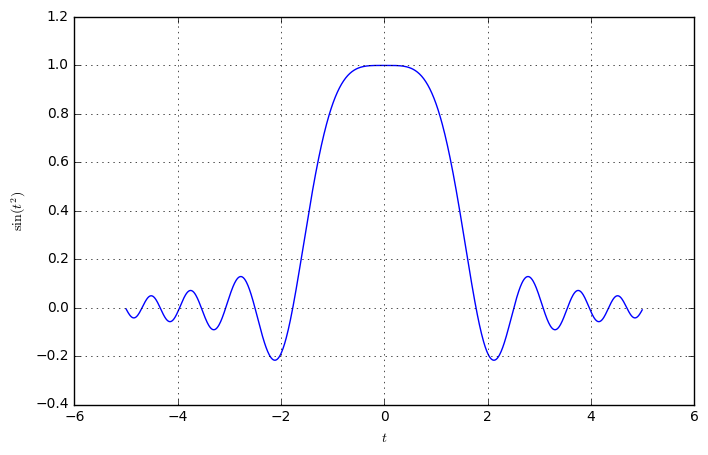# Welcome to DSPIllustrations.com!

I believe that digital signal processing and fundamentals of communications systems dont need to be taught on purely theoretic and mathematical level. There are so many things that can be easily visualized instead of being derived from tedious calculations.

This site is dedicated to illustrate fundamental aspects of signal processing and analysis with easy to follow code examples and graphical illustrations. This mixture of basic mathematical theory and direct illustration with source code allows to

• better understand the treatment,
• ensure correctness by numerical verification,
• learn, how equations can be modeled in computer programs.

Throughout the site, I use the Python programming language. Even though MATLAB is more popular among communication engineers, Python has the following particular advantages:

• Python is a general-purpose language, which has dedicated libraries to support signal processing. Hence, it is easier to structure your program and you're not lost, when you need to perform tasks that are not related to signal processing itself.
• Python supports literal programming with the use of Jupyter Notebooks. This makes it very suitable for explaining code with plain text and intermixed mathematical notation. This site makes heavy use of this feature and allows to download the documents directly to your own Jupyter Notebook to play around with the code examples.

Througout the page, some source code can be run interactively. Look out for the link below:Run code interactively  Hide

## Installing and setting up the Python development environment

I suggest installing a ready-made Python distribution, such as Anaconda Python. It contains all the packages that are necessary and is the simplest way to install Python. Just download the latest 64-Bit Python version and follow the instructions. Also, check this introduction for the Python installation Beginner's Guide

## Starting the Jupyter Notebook

Anaconda already installs the Jupyter Notebook including a shortcut for starting it. When you want to download the source code and load it into Jupyter, simply start the Jupyter Notebook, download the source code and save it to the folder where Jupyter is running. Try it with downloading the current script, by clicking Download Jupyter Notebook! on the right hand side!

# The sample notebook¶

This notebook is an example of how the Jupyter notebook works. You can download this notebook from the right-hand side, clicking Download Jupyter Notebook!, or you can directly add it to your running Jupyter notebook instance, by clicking Add Code to Jupyter Notebook.

Below are some version tests and some simple plotting functions.

Import some commonly needed packages.

import numpy as np
import scipy

import matplotlib.pyplot as plt
import matplotlib
%matplotlib inline
from matplotlib import animation
from IPython.display import HTML

from ipywidgets import interact
# workaround function for strange interact implementation
def showInInteract():
import inspect
for i in range(5):
if 'interaction.py' in inspect.stack()[i]: plt.show()


Check the versions of the different packagesRun code interactively  Hide
print ("Numpy version:      ", np.version.full_version)
print ("Scipy version:      ", scipy.version.full_version)
print ("Matplotlib version: ", matplotlib.__version__)

Numpy version:       1.11.3
Scipy version:       0.18.1
Matplotlib version:  1.5.3


Check the plotting functionality of Matplotlib. Here we show a simple static plot.Run code interactively  Hide
t = np.arange(-5, 5, 1/100)
plt.plot(t, np.sin(t*t)/(t*t))
plt.grid()
plt.xlabel('$t$')
plt.ylabel(r'$\sin(t^2)$');Define some function showing some chirps and inverse chirps. Then, animate it to show different parameters.Run code interactively  Hide
def showSin(f):
t = np.arange(-1, 1, 1/1000)
plt.plot(t, np.sin(2*np.pi*f*t*t))
plt.plot(t, np.cos(2*np.pi*f/(t**2+1)))
plt.text(0, 0.5, 'f=%.1f' % f, bbox=dict(facecolor='white'))